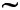# Similar Figures

### Basics

You have taken a nice photograph of an ancient monument and now you want to enlarge the size of your photograph from its original one. In the process of enlargement, it is ensured that the shape of the photograph remains the same from the original one, only the size differs. The size changes but the shape remains the same. By now, you have some cue about similarity.

Similar figures are geometrical figures, which have the same shape but not the same size.

Thus, two polygons of the same number of sides are similar, if

• their corresponding angles are equal
• their corresponding sides are in the same ratio

The symbol used to denote similarity between two figures is “### More Details

In your previous chapter, you studied about congruent figures. It is important to understand the difference between congruency and similarity. Â The two congruent figures have the same shape and size so that one can be superimposed on the other to cover it exactly and completely. However, in a pair of similar figures, the corresponding sides are in proportion but not equal. From the discussion, we can conclude that congruent figures are similar but similar figures are not congruent.

Criteria For Similarity of Two Triangles:

To prove similarity between two triangles it must fulfill two conditions

• their corresponding angles are equal
• their corresponding sides are in same ratio.Referring to the above figure:KLM =EFG,KML =EGF andLKM =FEG

KLÂ =Â KMÂ =Â LM
EF EG FG

Thus, we can say that the triangle KLM and triangle EFG are similar. Symbolically we write the above relation asKLMEFG because

It is important to note that, as in the case of congruency, similar triangles also should be written in the correct correspondence of their vertices i.e it would be incorrect to write triangle KLM is similar to triangle GFE.

It is important to note that if one polygon is similar to a second polygon and second polygon is similar to a third then the first polygon is also similar to the third polygon.

In similar figures since the corresponding angles are equal and the corresponding side are in the same ratio, if a side of a polygon is missing, we can find out using the ratio called proportion. Not only are the corresponding angles the same size in similar polygons, but also the sides are proportional. We can use the ratios to help the find missing values.In the above given figure PQRS and LMNO are two quadrilaterals with their corresponding angles equal. Thus,PSR =LON,PQR =LMN,QRS =MNO andSPQ =OLM, which proves that the two quadrilaterals are similar. As, the quadrilaterals are similar their sides must also be proportional.Â  Therefore, we can make proportions between the sides, in this wayIn the above figures, the measures of other lengths are given and we are supposed to find out the measure of segment MN. The length of MN can be found through proportion.

Since SR is to ON as OR is to MN we can write in this way:After substituting the values of the segment lengths, we get this proportion.After cross multiplying we get:

8n = 20

n = 20 = 2.5
8

Thus, MN = 2.5

### Examples:Referring to the above figure:

InABC,CAB = 180Â° – (30Â° +Â 100Â°) = 50Â° (As the sum of all the angles in a triangle equals 180Â°)

Thus,ABCPQR (As all the corresponding angles of both the triangles are equal)

Using, the proportions of the sides, we can say:Equating the proportions of two sides only, we see:By cross multiplying, we would get:

8PQ = 16

Thus, PQ = 16/8 = 2

See how well you understand the material – try our Similar Figures Test.